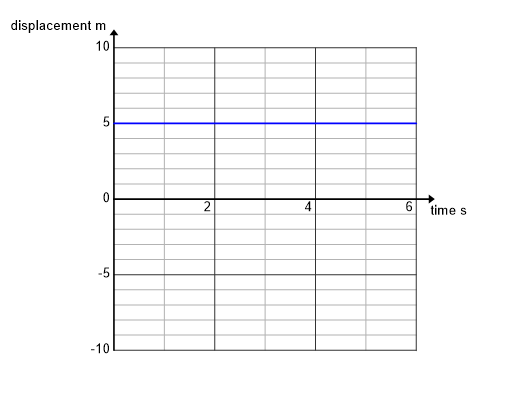Motion Graphs Quiz
A quiz to test your mastery of motion graphs
The final displacement of this object is
1 pointThe gradient of a displacement time graph is a measure of the
1 point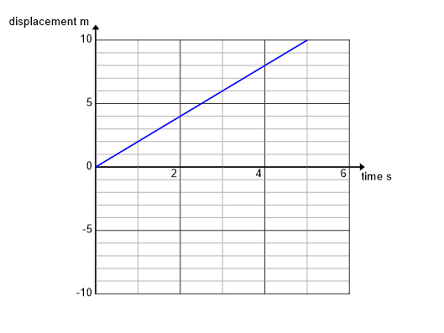A ball is thrown into the air and its velocity time graph is obtained. The maximum height the ball reached was
1 point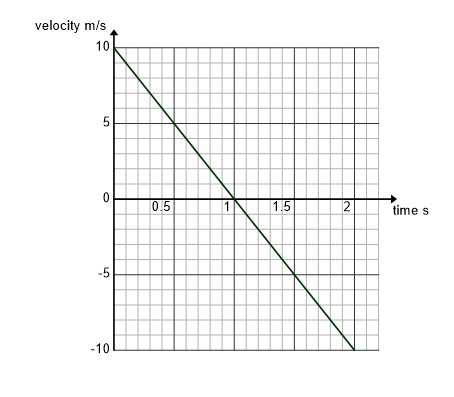The average velocity from 0 to 2 seconds of this motion is
1 point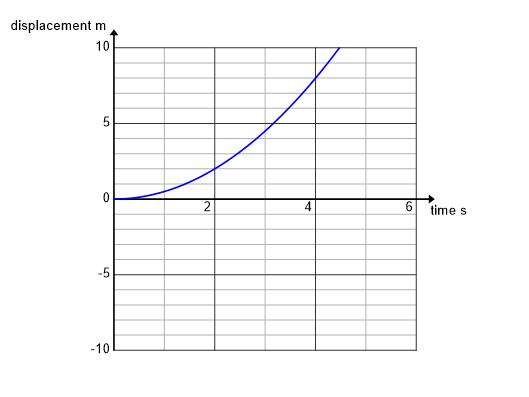This graph is a velocity time graph of a ball thrown into the air. The y axis is velocity measured in m/s and the x axis is time measured in s. The acceleration between 0 s and 0.8 s is
1 point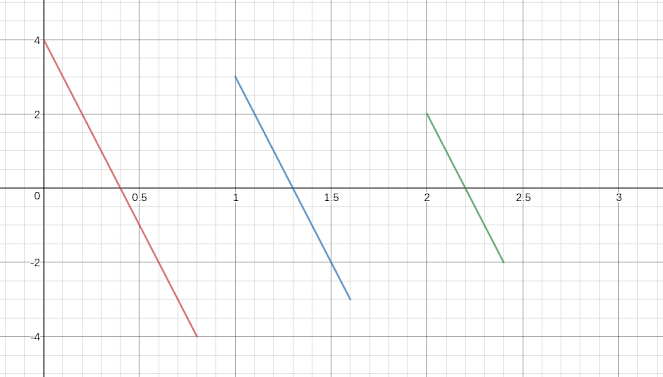This displacement graph shows an object
1 point